# Numerical Study on Unsteady Flow and Mass Transfer Past a Vertical Porous Plate with Variable Viscosity

Numerical Study on Unsteady Flow and Mass Transfer Past a Vertical Porous Plate with Variable Viscosity

Sharmin Sultana ShantaMd. Azmir Ibne Islam Kamonashis Mondol Sarder Firoz Ahmmed

Mathematics Discipline, Khulna University, Khulna 9208, Bangladesh

Corresponding Author Email:
sharmin3349@gmail.com
Page:
273-282
|
DOI:
https://doi.org/10.18280/mmep.070214
13 February 2020
|
Accepted:
17 May 2020
|
Published:
30 June 2020
| Citation

OPEN ACCESS

Abstract:

Present study is devoted for an unsteady flow mass and heat transfer past a vertical porous plate with variable viscosity. The effects of chemical reaction, heat source and thermal conductivity on the flow are also investigated. The partial differential equations, which are essentially nonlinear in nature, along with specific boundary conditions have been converted to the dimensionless form by using appropriate coordinate transformation. Explicit finite difference method has been applied to solve the dimensionless momentum, concentration and energy equations numerically. The velocity, concentration and temperature outlines have been discussed for well-known parameters whereas Nusselt number and skin friction have been represented in tabular forms. The variations of stream lines and isotherms have also been displayed graphically. Absolute conclusion has finally been drawn based on the numerical and graphical results.

Keywords:

explicit finite difference method, mass transfer, unsteady flow, variable viscosity, vertical porous plate

1. Introduction

Unsteady flow through an infinite vertical plate was explored by Uwanta et al. . Crank-Nicolson method was used to analyze the mass and heat transfer with the existence of thermal conductivity, chemical reaction and heat source parameter. Keeping all these thoughts in mind, we are fully motivated to focus on the effort done by Uwanta et al.  and want to examine the velocity, concentration and temperature outlines with variable viscosity. In other words, the objectives of this present research are to observe the effects of unsteady flow mass and heat transfer through a vertical porous plate along with thermal conductivity, chemical reaction, heat source and variable viscosity parameters. We are determined to apply an explicit finite difference method in order to obtain the numerical solutions of the governing equations. To be more specific, the problem formulation has been represented in Section 2. The numerical technique to solve the dimensionless equations is discussed in Section 3. In Section 4, numerical simulations with physical significance are reported graphically and the effects of various factors on velocity, concentration and temperature outlines are reflected. Moreover, the stream lines and isotherms have been displayed graphically whereas Nusselt number and skin friction have been demonstrated in tabular format. Section 5 finally contains the whole summary of our investigation.

2. Formulation of the Model

A two dimensional unsteady natural laminar convective flow of incompressible viscous, dissipative and radiating fluid (water or air) is considered along an isothermal infinite vertical porous plate. The acceleration due to the gravity (g) is assumed to be acted in downward direction. The $\bar{x}$-axis is considered to be directed upward along the vertical porous plate whereas $\bar{y}$-axis is always normal to the plate. We assume that the fluid and the plate are initially (i.e., at time$\bar{t}=0$) held at the similar temperature (${{\bar{T}}_{\infty }}$) and species concentration (${{\bar{C}}_{\infty }}$). The fluid starts moving along the vertical porous plate at $\bar{t}>0$, consequently, the temperature of the plate and the species concentration are elevated to ${{\bar{T}}_{w}}$ and ${{\bar{C}}_{w}}$ respectively which cause the differences both in temperature (${{\bar{T}}_{w}}-{{\bar{T}}_{\infty }}$) and in concentration (${{\bar{C}}_{w}}-{{\bar{C}}_{\infty }}$). Since the plate, in extent, is infinite, the physical variables are actually independent of $\bar{x}$ and are the functions of $\bar{t}\,$and$\bar{y}$. The variable viscosity, the nth-order chemical reaction and the variable thermal conductivity are assumed to be non-constant. It is also assumed that the thermal radiation is actually present as a form of a uni-directional flux along$\bar{y}$direction which means that the radiative heat flux (qr) is principally transverse with the vertical surface and can be expressed under Rosseland approximation as:

$\frac{\partial {{q}_{r}}}{\partial \overline{y}}=-\,4\sigma \,{{a}^{*}}\left( \overline{T}_{\infty }^{4}-{{\overline{T}}^{4}} \right)$

where, a* denotes the mean absorption effect for the thermal radiation constant, σ represents the Stefan-Boltzmann constant. It is our assumption that the differences in temperature within the flow are small enough, therefore we can expand ${{\overline{T}}^{4}}$in Taylor series about ${{\overline{T}}_{\infty }}$by neglecting the higher order terms as ${{\overline{T}}^{4}}\cong 4\overline{T}\overline{T}_{\infty }^{3}-3\overline{T}_{\infty }^{4}$.

Keeping Boussinesq approximation in mind, the governing equations such as continuity, momentum, concentration and energy along the velocity components $\bar{u}$ and $\bar{v}$ at $\bar{t}$in $\bar{x}$ and$\bar{y}$directions can be written as:

$\frac{\partial \overline{v}}{\partial \overline{y}}=0$        (1)

\begin{align} & \frac{\partial \overline{u}}{\partial \overline{t\,}}+\overline{v}\frac{\partial \overline{u}}{\partial \overline{y}}=\frac{1}{\rho }\frac{\partial }{\partial \overline{y}}\left( \mu \,(\overline{T})\frac{\partial \overline{u}}{\partial \overline{y}} \right)-\frac{\sigma {{B}_{\circ }}^{2}\overline{u}}{\rho }-\frac{\upsilon \overline{u}}{{{K}^{*}}} \\ & \,\,\,\,\,\,\,\,\,\,\,\,\,\,\,\,\,\,\,\,\,\,+g\beta (\overline{T}-{{\overline{T}}_{\infty }})+g{{\beta }^{*}}(\overline{C}-{{\overline{C}}_{\infty }})-b_{1}^{*}{{\overline{u}}^{2}} \\ \end{align}        (2)

$\frac{\partial \overline{C}}{\partial \overline{t\,}}+\overline{v}\frac{\partial \overline{C}}{\partial \overline{y}}=D\frac{{{\partial }^{2}}\overline{C}}{\partial {{\overline{y}}^{2}}}-{{R}^{*}}{{(\overline{C}-{{\overline{C}}_{\infty }})}^{n}}$                             (3)

\begin{align} & \frac{\partial \overline{T}}{\partial \overline{t\,}}+\overline{v}\frac{\partial \overline{T}}{\partial \overline{y}}=\frac{1}{\rho \,{{C}_{p}}}\frac{\partial }{\partial \overline{y}}\left( K(\overline{T})\frac{\partial \overline{T}}{\partial \overline{y}} \right)-\frac{1}{\rho {{C}_{p}}}\frac{\partial {{q}_{r}}}{\partial \overline{y}} \\ & \,\,\,\,\,\,\,\,\,\,\,\,\,\,\,\,\,\,\,\,\,\,\,\,\,\,+\frac{\upsilon }{{{C}_{p}}}{{\left( \frac{\partial \overline{u}}{\partial \overline{y}} \right)}^{2}}+{{b}^{*}}{{\overline{u}}^{2}}+\frac{Q}{\rho \,{{C}_{p}}}(\,\overline{T}-{{\overline{T}}_{\infty }}) \\ \end{align}          (4)

with the corresponding boundary conditions:

\left. \begin{align} & \overline{t\,}\le 0,\,\,\,\,\,\,\overline{u}=0,\,\,\,\overline{T}={{\overline{T}}_{\infty }},\,\,\,\overline{C}={{\overline{C}}_{\infty }}\,\,\,\,\,\,\,\,\,\,\text{for}\,\,\text{all}\,\,\,\overline{y} \\ & \overline{t\,}\,\,>0,\,\,\,\,\,\,\overline{u}=0,\,\,\,\overline{T}={{\overline{T}}_{w}},\,\,\,\overline{C}={{\overline{C}}_{w}}\,\,\,\,\,\,\,\,\,\,\text{at}\,\,\,\,\overline{y}=0\,\,\,\,\,\, \\ & \,\,\,\,\,\,\,\,\,\,\,\,\,\,\,\,\,\,\overline{u}\to 0,\,\,\overline{T}\to \,{{\overline{T}}_{\infty }},\,\,\overline{C}\to \,{{\overline{C}}_{\infty }}\,\,\,\,\,\,\,\text{as}\,\,\,\overline{y}\to \infty \\ \end{align} \right\}\,         (5)

From Eq. (1), we understand that suction velocity may take the form of a constant or a function of time. By integrating Eq. (1), suction velocity is assumed as $\overline{v}=-\,{{v}_{0}}$which is perpendicular to the plate where v0 represents the scale of suction velocity. Here, negative sign specifies the phenomena that suction is in the direction of the plate. Also, ${{v}_{0}}>0$is corresponding to the steady suction velocity perpendicular to the surface.

Now we apply the following dimensionless quantities:

\begin{align} & U=\frac{\overline{u}}{{{U}_{\circ }}},\,\,\,y=\frac{\overline{y}\,{{U}_{\circ }}}{\upsilon },\,\,\,t=\frac{\overline{t\,}\,{{U}_{\circ }}^{2}}{\upsilon },\,\,\,\, \\ & \theta =\frac{\overline{T}-{{\overline{T}}_{\infty }}}{{{\overline{T}}_{w}}-{{\overline{T}}_{\infty }}},\,\,\,C=\frac{\overline{C}-{{\overline{C}}_{\infty }}}{{{\overline{C}}_{w}}-{{\overline{C}}_{\infty }}},\,\,\,\,b=\frac{{{b}^{*}}\upsilon }{{{\overline{T}}_{w}}-{{\overline{T}}_{\infty }}},\,\,\,\, \\ & Sc=\frac{\upsilon }{D},\,\,\,M=\frac{\upsilon \sigma B_{\circ }^{2}}{\rho U_{\circ }^{2}},\,\,\,\alpha =\frac{{{\upsilon }_{\circ }}}{{{U}_{\circ }}},\,\,\,{{b}_{1}}=\frac{b_{1}^{*}\upsilon }{{{U}_{\circ }}},\,\, \\ & K=\frac{{{K}^{*}}U_{\circ }^{2}}{{{\upsilon }^{2}}},\,\,\,\,N=\frac{16{{a}^{*}}\sigma \overline{T}_{\infty }^{3}{{\upsilon }^{2}}}{{{k}_{\circ }}{{U}_{\circ }}^{2}},\,\,\,\Pr =\frac{\upsilon \rho {{C}_{p}}}{{{k}_{\circ }}},\,\,\,\, \\ & Ec=\frac{U_{\circ }^{2}}{{{C}_{p}}({{\overline{T}}_{w}}-{{\overline{T}}_{\infty }})},\,\,\,\,Gr=\frac{g\beta \upsilon \,({{\overline{T}}_{w}}-{{\overline{T}}_{\infty }})}{U_{\circ }^{3}},\,\,\, \\ & Gc=\frac{g{{\beta }^{*}}\upsilon \,({{\overline{C}}_{w}}-{{\overline{C}}_{\infty }})}{U_{\circ }^{3}},\,\,\upsilon =\frac{{{\mu }_{0}}}{\rho } \\ \end{align}              (6)

We now use the dimensional variable thermal conductivity:

$K(\overline{T})={{k}_{\text{o}}}(1+{{\gamma }_{10}}(\,\overline{T}-{{\overline{T}}_{\infty }})\,)$

which depends on the temperature. Here, γ10 is any constant and ko is the thermal conductivity of ambient fluid. Let τ be the dimensionless variable thermal conductivity which can be written as$\,\tau ={{\gamma }_{10}}({{\overline{T}}_{w}}-{{\overline{T}}_{\infty }})$. Therefore, $K(\overline{T})={{k}_{\text{o}}}(1+\tau \theta \,)$.

We also incorporate the dimensional variable viscosity:

$\mu \,(\overline{T})={{\mu }_{\text{o}}}(1+{{\gamma }_{20}}(\,\overline{T}-{{\overline{T}}_{\infty }})\,)$

Here, γ20 is any constant and μo is the variable viscosity of ambient fluid. Let γ is the dimensionless variable viscosity which may be defined as $\gamma ={{\gamma }_{20}}(\,{{\overline{T}}_{w}}-{{\overline{T}}_{\infty }})$.

Therefore, $\mu \,(\overline{T})={{\mu }_{0}}\,(\,1+\gamma \,\theta \,)$.

We also take into account the dimensionless chemical reaction parameter:

$Kr=\frac{\upsilon {{R}^{*}}{{({{\overline{C}}_{w}}-{{\overline{C}}_{\infty }})}^{n-1}}}{U_{\circ }^{2}}$

where, R* is representing the dimensional chemical reaction and n is denoting the reaction order.

A dimensionless heat source parameter S to provide the temperature is introduced as:

$S=\frac{Q{{\upsilon }^{2}}}{{{k}_{\circ }}{{U}_{\circ }}^{2}}\,$

where, Q denotes the volumetric rate of heat source.

Finally by applying the dimensionless quantities, Eqns. (1)-(4) reduce to:

\begin{align} & \left( \frac{\partial U}{\partial t}-\alpha \frac{\partial U}{\partial y} \right)=(1+\gamma \,\theta )\frac{{{\partial }^{2}}U}{\partial {{y}^{2}}}+\gamma \,\left( \frac{\partial \theta }{\partial y} \right)\,\left( \frac{\partial U}{\partial y} \right) \\ & \,\,\,\,\,\,\,\,\,\,\,\,\,\,\,\,\,\,\,\,\,\,\,\,\,\,\,\,\,\,\,\,\,\,-\left( M-\frac{1}{k} \right)U+Gr\theta +GcC-{{b}_{1}}{{U}^{2}} \\ \end{align}          (7)

$\frac{\partial C}{\partial t}-\alpha \frac{\partial C}{\partial y}=\frac{1}{Sc}\frac{{{\partial }^{2}}C}{\partial {{y}^{2}}}-Kr{{C}^{n}}$         (8)

\begin{align} & \frac{\partial \theta }{\partial t}-\alpha \frac{\partial \theta }{\partial y}=\frac{(1+\tau \theta )}{\Pr }\frac{{{\partial }^{2}}\theta }{\partial {{y}^{2}}}+{{\left( \frac{\partial \theta }{\partial y} \right)}^{2}}\frac{\tau }{\Pr } \\ & \,\,\,\,\,\,\,\,\,\,\,\,\,\,\,\,\,\,\,\,\,\,\,\,\,\,\,\,\,\,\,\,\,\,\,\,\,\,\,\,+\frac{\left( S-N \right)}{\Pr }\theta +Ec\,{{\left( \frac{\partial U}{\partial y} \right)}^{2}}+b{{U}^{2}} \\ \end{align}      (9)

The boundary conditions for the problem are given below:

\left. \begin{align} & \,t\le 0,\,\,\,\,\,\,\,\,U=0,\,\,\,\theta =0,\,\,\,C=0\,\,\,\,\,\,\,\,\,\,\,\,\,\,\text{for}\,\,\text{all}\,\,y\,\,\,\,\, \\ & \,t>0,\,\,\,\,\,\,\,\,U=0,\,\,\,\theta =1,\,\,\,C=1\,\,\,\,\,\,\,\,\,\,\,\,\,\,\,\,at\,\,y=0\,\, \\ & \,\,\,\,\,\,\,\,\,\,\,\,\,\,\,\,\,\,\,\,U\to 0,\,\,\,\theta \to 0,\,\,\,C\to 0\,\,\,\,\,\,\,as\,\,y\to \infty \\ \end{align} \right\}            (10)

The physical quantities, i.e., Nusselt number (Nu) and skin friction (Cf ) can be written in dimensionless form as follows:

$Nu=\frac{1}{\sqrt{2}}G{{r}^{-\,\frac{3}{4}}}{{\left( \frac{\partial \theta }{\partial y} \right)}_{y=0}}$ and ${{C}_{f}}=-\frac{1}{2\sqrt{2}}G{{r}^{-\,\frac{3}{4}}}{{\left( \frac{\partial U}{\partial y} \right)}_{y=0}}$

3. Numerical Procedure

Explicit finite difference method has been applied to obtain the solution of the Eqns. (7)-(9) with boundary conditions Eq. (10). The boundary layer area is subdivided by perpendicular lines generated from y-axis. Figure 1 displays the grid space by considering the maximum height of the plate: xmax = 20, maximum height of the boundary layer: ymax= 50, grid spacing: m1 = 100, n1 = 200 with ∆x = 0.20 (0 ≤ x ≤ 20) and ∆y = 0.25 (0 ≤ y ≤ 50). A small time step ∆t is taken as 0.005.

## 1.png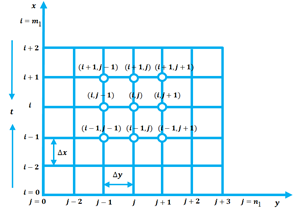Figure 1. Grid space based on finite difference

Now we define U, C and θ in the finite difference form:

${{\left( \frac{\partial U}{\partial t} \right)}_{i,\,\,j}}=\frac{{{U}_{new}}-U_{i,\,\,j}^{{}}}{\Delta t},$

${{\left( \frac{{{\partial }^{2}}U}{\partial {{y}^{2}}} \right)}_{i,\,\,j}}=\frac{{{U}_{i,\,\,j+1}}+{{U}_{i,\,\,j-1}}-2{{U}_{i,\,\,j}}}{{{(\Delta y)}^{2}}},$

${{\left( \frac{\partial C}{\partial t} \right)}_{i,\,\,j}}=\frac{{{C}_{new}}-C_{i,\,\,j}^{{}}}{\Delta t},$   ${{\left( \frac{\partial C}{\partial y} \right)}_{i,\,\,j}}=\frac{C_{i,\,\,j+1}^{{}}-C_{i,\,\,j}^{{}}}{\Delta y},$

${{\left( \frac{{{\partial }^{2}}C}{\partial {{y}^{2}}} \right)}_{i,\,\,j}}=\frac{{{C}_{i,\,\,j+1}}+{{C}_{i,\,\,j-1}}-2{{C}_{i,\,\,j}}}{{{(\Delta y)}^{2}}},$

${{\left( \frac{\partial \theta }{\partial t} \right)}_{i,\,\,j}}=\frac{{{\theta }_{new}}-\theta _{i,\,\,j}^{{}}}{\Delta t},$         ${{\left( \frac{\partial \theta }{\partial y} \right)}_{i,\,\,j}}=\frac{\theta _{i,\,\,j+1}^{{}}-\theta _{i,\,\,j}^{{}}}{\Delta y},$

${{\left( \frac{{{\partial }^{2}}\theta }{\partial {{y}^{2}}} \right)}_{i,\,\,j}}=\frac{{{\theta }_{i,\,\,j+1}}+{{\theta }_{i,\,\,j-1}}-2{{\theta }_{i,\,\,j}}}{{{(\Delta y)}^{2}}}.$

Using the above forms in Eqns. (7)-(10), we get a suitable set of equations as:

\begin{align} & \frac{{{U}_{new}}-U_{i,\,\,j}^{{}}}{\Delta t}-\alpha \frac{U_{i,\,\,j+1}^{{}}-U_{i,\,\,j}^{{}}}{\Delta y} \\ & \,\,\,\,\,\,\,\,\,\,\,\,\,=(1+\gamma \,\theta _{i\,,\,j}^{{}})\frac{{{U}_{i,\,\,j+1}}+{{U}_{i,\,\,j-1}}-2{{U}_{i,\,\,j}}}{{{(\Delta y)}^{2}}}\, \\ & \,\,\,\,\,\,\,\,\,\,\,\,\,\,\,\,\,\,\,\,+\gamma \,\frac{\theta _{i,\,\,j+1}^{{}}-\theta _{i,\,\,j}^{{}}}{\Delta y}\frac{U_{i,\,\,j+1}^{{}}-U_{i,\,\,j}^{{}}}{\Delta y}\,-\left( M-\frac{1}{k} \right)U_{i,\,\,j}^{{}} \\ & \,\,\,\,\,\,\,\,\,\,\,\,\,\,\,\,\,\,\,\,\,\,\,\,\,\,\,\,\,\,\,\,\,\,\,\,\,\,\,\,\,\,\,\,\,\,\,\,\,+Gr\theta _{i,\,\,j}^{{}}+GcC_{i,\,\,j}^{{}}-{{b}_{1}}{{(U_{i,\,\,j}^{{}})}^{2}} \\ \end{align}            (11)

\begin{align} & \frac{{{C}_{new}}-C_{i,\,\,j}^{{}}}{\Delta t}-\alpha \frac{C_{i,\,\,j+1}^{{}}-C_{i,\,\,j}^{{}}}{\Delta y} \\ & \,\,\,\,\,\,\,\,\,\,\,\,\,\,\,\,\,\,\,=\frac{1}{Sc}\frac{{{C}_{i,\,\,j+1}}+{{C}_{i,\,\,j-1}}-2{{C}_{i,\,\,j}}}{{{(\Delta y)}^{2}}}-Kr{{(C_{i,\,\,j}^{{}})}^{n}} \\ \end{align}             (12)

\begin{align} & \frac{{{\theta }_{new}}-\theta _{i,\,\,j}^{{}}}{\Delta t}-\alpha \frac{\theta _{i,\,\,j+1}^{{}}-\theta _{i,\,\,j}^{{}}}{\Delta y} \\ & =\frac{(\tau \,\theta _{i\,,\,j}^{{}}+1)}{\Pr }\frac{{{\theta }_{i,\,\,j+1}}+{{\theta }_{i,\,\,j-1}}-2{{\theta }_{i,\,\,j}}}{{{(\Delta y)}^{2}}}+{{\left( \frac{\theta _{i,\,\,j+1}^{{}}-\theta _{i,\,\,j}^{{}}}{\Delta y} \right)}^{2}}\frac{\tau }{\Pr } \\ & \,\,\,\,\,\,\,\,\,\,\,+\frac{(S-N)}{\Pr }\theta _{i,\,\,j}^{{}}+Ec\,{{\left( \frac{U_{i,\,\,j+1}^{{}}-U_{i,\,\,j}^{{}}}{\Delta y} \right)}^{2}}+b{{(U_{i,\,\,j}^{{}})}^{2}} \\ \end{align}              (13)

subject to the boundary conditions:

\left. \begin{align} & \,t>0,\,\,\,\,U_{i\,,\,j}^{0}=0,\,\,\theta _{i\,,\,j}^{0}=1,\,\,C_{i\,,\,j}^{0}=1\,\,\,\,\,\,\,\,\,\,\,when\,\,y=0\,\,\, \\ & \,\,\,\,\,\,\,\,\,\,\,\,\,\,\,U_{i\,,\,j}^{n}\to 0,\,\,\theta _{i\,,\,j}^{n}\to 0,\,\,C_{i\,,\,j}^{n}\to 0\,\,\,\,when\,\,y\to \infty \, \\ \end{align} \right\}\,               (14)

4. Results and Discussion

The dimensionless velocity, concentration and temperature outlines are discussed numerically with governing parameters: thermal Grashof number (Gr), mass Grashof number (Gc), suction parameter (α), variable thermal conductivity (τ), variable viscosity parameter (γ), inertia number (b1), hall current parameter (b), chemical reaction parameter (Kr), porosity (K), magnetic field parameter (M), Schmidt number (Sc), heat source parameter (S), Prandtl number (Pr), Eckert number (Ec) and finally thermal radiation parameter (N). The constant values of these parameters have been provided in Table 1. The variations of velocity outlines have been shown from Figures 2-8. Temperature outlines are represented from Figures 9-13 whereas Figures 14-16 represent the concentration outlines. Stream lines along with isotherms have been plotted and represented in Figures 17-20.

Table 1. Constant values of the parameters

 Parameters Gr Gc α τ γ b1 b Kr Values 1.0 1.0 3.0 0.1 0.25 1.0 1.0 0.1 Parameters K M Sc S Pr Ec N Values 0.1 1.0 0.22 0.1 1.0 0.01 1.0

Figure 2 displays the velocity outlines for variable viscosity parameter (γ). Since viscosity is responsible for flow resistance, we see that velocity decreases when viscosity increases. Velocity peaks decrease about 3.33%, 6.67% and 7.33% when γ is changed from its base value 0.25 to 0.50, 0.75 and 1.0 respectively.

Figure 3 represents that velocity outlines decrease significantly with the increase of suction parameter (α). When α changes from 3.0 to 5.0, velocity peak declines about 35.53%. Similarly, peak value declines near about 52.63% (when α = 7.0) and 63.16% (when α = 9.0) compared to the peak value found for the baseline of α. From Figure 4, it has been observed that velocity outlines increase with the increase of thermal Grashof number (Gr).

Figure 5 also shows the similar result, i.e., velocity outlines increase when the value of the mass Grashof number (Gc) is increased. We observe from Figure 6 that velocity outlines show declining effects when the inertia number (b1) is increased. Peak magnitudes decrease to 10.14%, 18.12% and 24.64% when b1 is increased from 1.0 to 15.0, 30.0 and 45.0 respectively. This phenomenon occurs, i.e., the velocity of the fluid tends to decrease, due to the air resistance and friction.

## 2.pngFigure 2. Velocity outlines for γ

## 3.png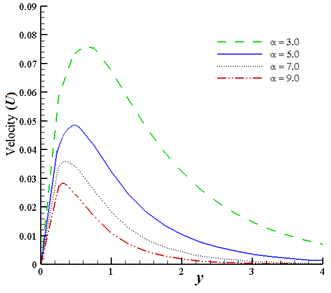Figure 3. Velocity outlines for α

## 4.png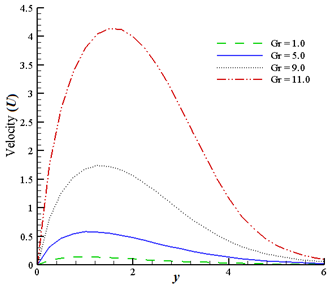Figure 4. Velocity outlines for Gr

## 5.png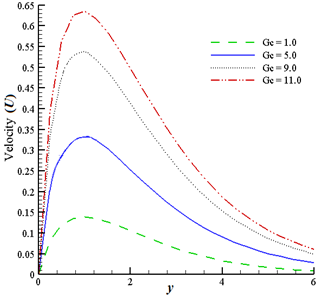Figure 5. Velocity outlines for Gc

## 6.png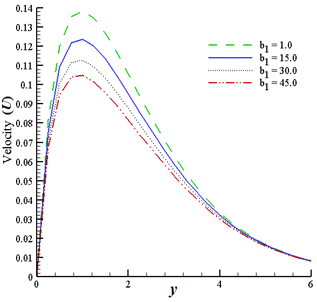Figure 6. Velocity outlines for b1

## 7.png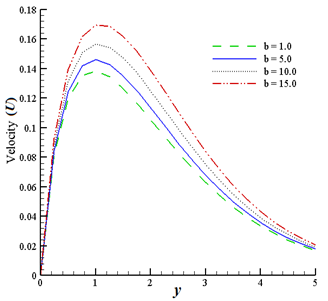Figure 7. Velocity outlines for b

Figure 7 represents the velocity outlines with respect to the hall current parameter (b). We understand that velocity distributions rise to 5.07%, 12.32% and 23.18% with the increase of the parameter b from 1.0 to 5.0, 10.0 and 15.0 respectively. Due to the hall current, an electric field is experienced as a result of which, fluid velocity increases. From Figure 8, it is found that the velocity outlines decrease with the increase of the magnetic field parameter (M). This happens because larger values of M generate a drag force or Lorentz force which competes against the motion of the fluid. Figure 9 shows that the temperature outlines increase when the values of the heat source parameter (S) are increased (i.e., S = 0.1, 0.4, 0.7, 1.0). Temperature distribution for variable thermal conductivity (τ) is shown in Figure 10. We understand that compared to the baseline value of τ (i.e., τ = 0.1), temperature outlines increase about 16% (for τ = 0.7), 40% (for τ = 1.4) and 60% (for τ = 2.1). Variations in temperature outlines for multiple values of Prandtl number (Pr = 1.0, 2.0, 2.2, 7.0) have been represented in Figure 11, from where it has been perceived that temperature outlines decrease while Pr is increased. Similar result is seen from Figure 12 where the temperature distribution is represented for α = 3.0, 5.0, 7.0 and 9.0.

## 8.png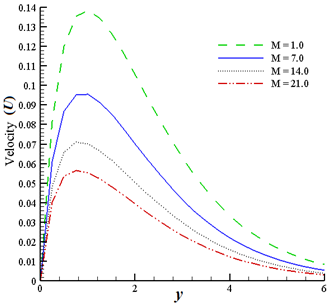Figure 8. Velocity outlines for M

## 9.png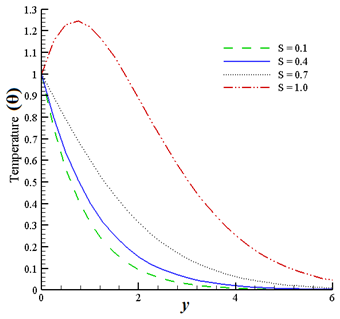Figure 9. Temperature outlines for S

Figure 13 demonstrates the temperature outlines for thermal radiation parameter N = 1.0, 7.0, 14.0 and 21.0. We observe that temperature outlines decrease significantly when N is increased. From Figure 14, we notice that concentration outlines tend to decrease with the increase of chemical reaction parameter (Kr). Concentration outlines decrease to 25%, 79.31% and 89.66% when Kr is increased to 1.5, 15.0 and 25.0 respectively from its baseline value.

## 10.png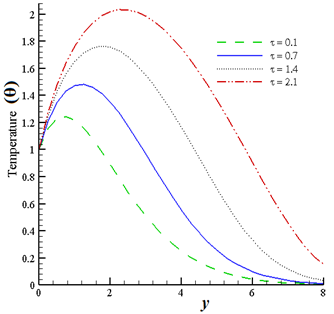Figure 10. Temperature outlines for τ

## 11.png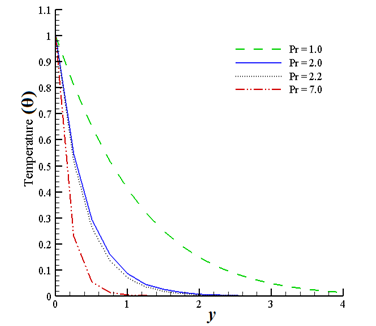Figure 11. Temperature outlines for Pr

## 12.pngFigure 12. Temperature outlines for α

## 13.pngFigure 13. Temperature outlines for N

## 14.png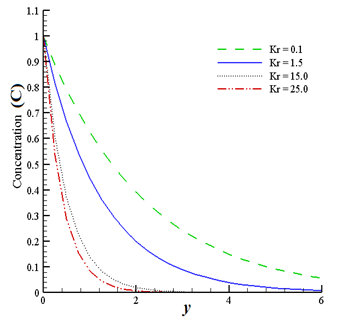Figure 14. Concentration outlines for Kr

## 15.png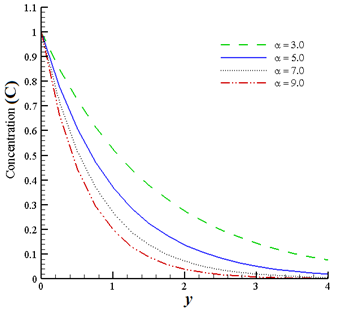Figure 15. Concentration outlines for α

Figure 15 displays that concentration outlines decline due to the increase of α. Similar effects can be observed from Figure 16, that means, concentration outlines drop with the increase of Schmidt number (Sc). Figure 17 and Figure 18 represent the streamlines aimed at γ = 0.25 and γ = 0.50 respectively. We see that the streamlines increase with the increase of variable viscosity which has an effect on momentum along with thermal boundary layers. Isotherms are displayed in Figures 19-20 with respect to γ. It is revealed that isotherms show declining effects when γ is changed from 0.25 to 0.50. The variation of Nusselt number (Nu) has been shown in Table 2 for Gr, Gc, α, τ, γ, b1, b, Kr, K, M, Sc, S, Pr, Ec and N. It is observed that Nusselt number shows decreasing effect for Gr, Gc, τ, b, K and Ec whereas increasing effect is found for α, γ, b1, Kr, M, Sc, S, Pr and N. Table 3 shows the variation of skin friction (Cf ) for Gr, Gc, α, τ, γ, b1, b, Kr, K, M, Sc, S, Pr, Ec and N. It is understood that skin friction decreases for Gr, α, b1, b, Kr, M, Sc, S, Pr and N whereas it increases for Gc, τ, γ, K and Ec.

Table 2. Summary of Nusselt number (Nu)

 Gr Gc α τ γ b1 b Kr K M Sc S Pr Ec N Nu 1.0 1.0 3.0 0.1 0.25 1.0 1.0 0.1 0.1 1.0 0.22 0.1 1.0 0.01 1.0 0.93630 5.0 1.0 3.0 0.1 0.25 1.0 1.0 0.1 0.1 1.0 0.22 0.1 1.0 0.01 1.0 0.26673 1.0 5.0 3.0 0.1 0.25 1.0 1.0 0.1 0.1 1.0 0.22 0.1 1.0 0.01 1.0 0.86094 1.0 1.0 5.0 0.1 0.25 1.0 1.0 0.1 0.1 1.0 0.22 0.1 1.0 0.01 1.0 1.65592 1.0 1.0 3.0 0.7 0.25 1.0 1.0 0.1 0.1 1.0 0.22 0.1 1.0 0.01 1.0 0.58335 1.0 1.0 3.0 0.1 0.50 1.0 1.0 0.1 0.1 1.0 0.22 0.1 1.0 0.01 1.0 0.93649 1.0 1.0 3.0 0.1 0.25 15.0 1.0 0.1 0.1 1.0 0.22 0.1 1.0 0.01 1.0 0.93709 1.0 1.0 3.0 0.1 0.25 1.0 5.0 0.1 0.1 1.0 0.22 0.1 1.0 0.01 1.0 0.90672 1.0 1.0 3.0 0.1 0.25 1.0 1.0 1.5 0.1 1.0 0.22 0.1 1.0 0.01 1.0 0.93763 1.0 1.0 3.0 0.1 0.25 1.0 1.0 0.1 1.0 1.0 0.22 0.1 1.0 0.01 1.0 0.89995 1.0 1.0 3.0 0.1 0.25 1.0 1.0 0.1 0.1 7.0 0.22 0.1 1.0 0.01 1.0 0.93984 1.0 1.0 3.0 0.1 0.25 1.0 1.0 0.1 0.1 1.0 0.60 0.1 1.0 0.01 1.0 0.93943 1.0 1.0 3.0 0.1 0.25 1.0 1.0 0.1 0.1 1.0 0.22 0.4 1.0 0.01 1.0 1.46162 1.0 1.0 3.0 0.1 0.25 1.0 1.0 0.1 0.1 1.0 0.22 0.1 2.0 0.01 1.0 3.22484 1.0 1.0 3.0 0.1 0.25 1.0 1.0 0.1 0.1 1.0 0.22 0.1 1.0 0.05 1.0 0.89884 1.0 1.0 3.0 0.1 0.25 1.0 1.0 0.1 0.1 1.0 0.22 0.1 1.0 0.01 7.0 0.93741

Table 3. Summary of skin friction (Cf )

 Gr Gc α τ γ b1 b Kr K M Sc S Pr Ec N Cf 1.0 1.0 3.0 0.1 0.25 1.0 1.0 0.1 0.1 1.0 0.22 0.1 1.0 0.01 1.0 0.30397 5.0 1.0 3.0 0.1 0.25 1.0 1.0 0.1 0.1 1.0 0.22 0.1 1.0 0.01 1.0 0.26404 1.0 5.0 3.0 0.1 0.25 1.0 1.0 0.1 0.1 1.0 0.22 0.1 1.0 0.01 1.0 0.93534 1.0 1.0 5.0 0.1 0.25 1.0 1.0 0.1 0.1 1.0 0.22 0.1 1.0 0.01 1.0 0.27272 1.0 1.0 3.0 0.7 0.25 1.0 1.0 0.1 0.1 1.0 0.22 0.1 1.0 0.01 1.0 0.31834 1.0 1.0 3.0 0.1 0.50 1.0 1.0 0.1 0.1 1.0 0.22 0.1 1.0 0.01 1.0 0.30401 1.0 1.0 3.0 0.1 0.25 15.0 1.0 0.1 0.1 1.0 0.22 0.1 1.0 0.01 1.0 0.28819 1.0 1.0 3.0 0.1 0.25 1.0 5.0 0.1 0.1 1.0 0.22 0.1 1.0 0.01 1.0 0.30385 1.0 1.0 3.0 0.1 0.25 1.0 1.0 1.5 0.1 1.0 0.22 0.1 1.0 0.01 1.0 0.28861 1.0 1.0 3.0 0.1 0.25 1.0 1.0 0.1 1.0 1.0 0.22 0.1 1.0 0.01 1.0 0.64297 1.0 1.0 3.0 0.1 0.25 1.0 1.0 0.1 0.1 7.0 0.22 0.1 1.0 0.01 1.0 0.23332 1.0 1.0 3.0 0.1 0.25 1.0 1.0 0.1 0.1 1.0 0.60 0.1 1.0 0.01 1.0 0.26901 1.0 1.0 3.0 0.1 0.25 1.0 1.0 0.1 0.1 1.0 0.22 0.4 1.0 0.01 1.0 0.28745 1.0 1.0 3.0 0.1 0.25 1.0 1.0 0.1 0.1 1.0 0.22 0.1 2.0 0.01 1.0 0.23839 1.0 1.0 3.0 0.1 0.25 1.0 1.0 0.1 0.1 1.0 0.22 0.1 1.0 0.05 1.0 0.62303 1.0 1.0 3.0 0.1 0.25 1.0 1.0 0.1 0.1 1.0 0.22 0.1 1.0 0.01 7.0 0.28693

## 16.pngFigure 16. Concentration outlines for Sc

## 17.png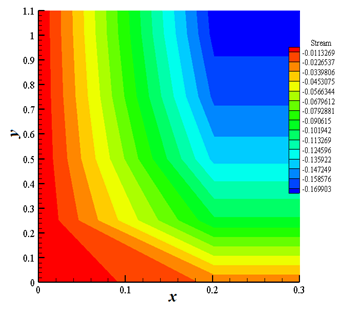Figure 17. Streamlines for γ = 0.25

## 18.png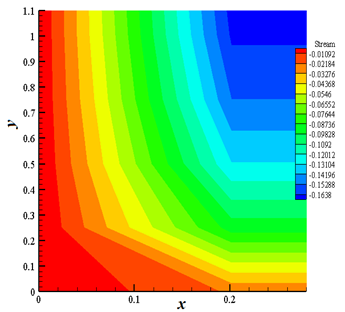Figure 18. Streamlines for γ = 0.50

## 19.png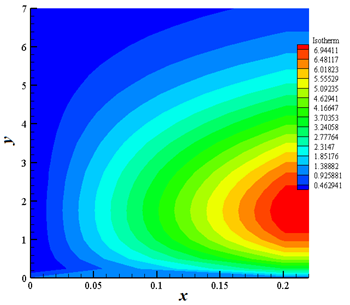Figure 19. Isotherms for γ = 0.25

## 20.png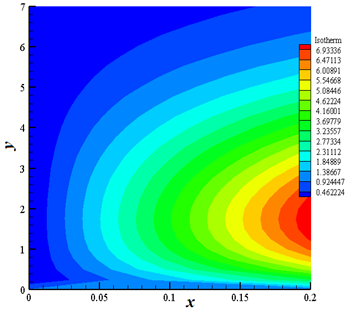Figure 20. Isotherms for γ = 0.50

5. Conclusions

In this paper, a two dimensional unsteady flow through a vertical porous plate with viscosity, chemical reaction, heat source and thermal conductivity has been discussed. The current analysis provides important insights in understanding complex flow patterns based on specific physical parameters and reveals the following scenarios:

• Velocity outlines show an increasing behavior with the increase of mass Grashof number, thermal Grashof number and hall current whereas an opposite phenomenon, that is, decreasing effect is observed with the increase of variable viscosity, suction parameter, inertia number and magnetic field parameter.
• Temperature outlines increase with the intensification of heat source parameter and variable thermal conductivity. While on the other hand, temperature outlines show decreasing effects due to the augmentation of Prandtl number, suction parameter and thermal radiation parameter.
• Concentration outlines tend to decline with the increase of suction parameter, chemical reaction and Schmidt number.
• Nusselt number mainly increases with the increase of variable viscosity, chemical reaction, heat source, suction parameter, inertia number, magnetic field, Schmidt number, Prandtl number and thermal radiation parameter whereas it decreases with the increase of variable thermal conductivity, mass Grashof number, thermal Grashof number, hall current, porosity and Eckert number.
• Skin friction shows an increasing effect due to the increase of variable viscosity, variable thermal conductivity, mass Grashof number, porosity and Eckert number. Conversely, it displays decreasing effects due to the increase of chemical reaction, heat source, thermal Grashof number, suction parameter, inertia number, hall current, magnetic field, Schmidt number, Prandtl number and thermal radiation parameter.
Acknowledgment

The authors are grateful to the anonymous reviewers and the editor for their constructive comments and suggestions which have significantly improved the quality of the paper.

Nomenclature
 a* Mean absorption effect b Dimensionless hall current b* Dimensional hall current b1 Inertia number $b_{1}^{*}$ Forchheimer parameter Bo Magnetic field intensity C Dimensionless mass concentration Cf Skin friction Cp Specific heat at constant pressure $\bar{C}$ Mass concentration $\bar{C}_{w}$ Species concentration at plate surface $\bar{C}_{\infty}$ Concentration for free stream D Chemical molecular diffusivity Ec Eckert number g Acceleration due to the gravity Gc Mass Grashof number Gr Thermal Grashof number ko Thermal conductivity of ambient fluid K * Permeability K Porosity $K(\bar{T})$ Dimensional variable thermal conductivity Kr Dimensionless chemical reaction M Magnetic field n Reaction order N Thermal radiation parameter Nu Nusselt number Pr Prandtl number qr Radiative heat flux R* Dimensional chemical reaction Q Volumetric rate of heat source S Dimensionless heat source parameter Sc Schmidt number $\bar{t}$ Time t Time used for dimensionless $\bar{T}$ Temperature $\bar{T}_{w}$ Wall temperature $\bar{T}_{\infty}$ Free stream temperature $\bar{u}, \bar{v}$ Velocity components along $\bar{x}$ and $\bar{y}$ directions U Dimensionless velocity component in x direction Uo Uniform velocity Greek symbols α Suction parameter β Thermal expansion coefficient β* Concentration expansion coefficient γ Dimensionless variable viscosity υ Kinematic viscosity τ Dimensionless variable thermal conductivity ρ Density σ Stefan-Boltzmann constant θ Dimensionless temperature $\mu(\bar{T})$ Dimensional variable viscosity μo Variable viscosity of ambient fluid
References

 Ahmed, R., Uddin, R., Rana, B.M.J., Selim, R., Ahmmed, S.F. (2016). Study on periodic MHD flow with temperature dependent viscosity and thermal conductivity past an isothermal oscillating cylinder. World Journal of Mechanics, 6(11): 419-440. http://dx.doi.org/10.4236/wjm.2016.611030

 Ali, M., Alam, M.S. (2014). Study on MHD boundary layer flow of combined heat and mass transfer over a moving inclined plate in a porous medium with suction and viscous dissipation in presence of hall effect. Engineering International, 2(1): 43-62. https://doi.org/10.18034/ei.v2i1.207

 Arifuzzaman, S.M., Khan, M.S., Mehedi, M.F.U., Rana B.M.J., Ahmmed, S.F. (2018). Chemically reactive and naturally convective high speed MHD fluid flow through an oscillatory vertical porous plate with heat and radiation absorption effect. Engineering Science and Technology, an International Journal, 21(2): 215-228. https://doi.org/10.1016/j.jestch.2018.03.004

 Aurangzaib, Kasim, A.R.M., Mohammad, N.F., Shafie, S. (2013). Unsteady MHD mixed convection flow with heat and mass transfer over a vertical plate in a micropolar fluid-saturated porous medium. Journal of Applied Science and Engineering, 16(2): 141-150. https://doi.org/10.6180/jase.2013.16.2.05

 Devi, S.P.A., Raj, J.W.S. (2014). Numerical simulation of magneto hydrodynamic forced convective boundary layer flow past a stretching/shrinking sheet prescribed with variable heat flux in the presence of heat source and constant suction. Journal of Applied Fluid Mechanics, 7(3): 415-423. https://doi.org/10.36884/jafm.7.03.19411

 Ghara, N., Das, S., Maji, S.L., Jana, R.N. (2012). Effect of radiation on MHD free convection flow past an impulsively moving vertical plate with ramped wall temperature. American Journal of Scientific and Industrial Research, 3(6): 376-386. https://doi.org/10.5251/ajsir.2012.3.6.376.386

 Hayat, T., Ashraf, M.B., Shehzad, S.A., Alsaedi, A. (2015). Mixed convection flow of Casson nanofluid over a stretching sheet with convectively heated chemical reaction and heat source/sink. Journal of Applied Fluid Mechanics, 8(4): 803-813. https://doi.org/10.18869/acadpub.jafm.67.223.22995

 Hossain, M.A., Mondal, R.K., Ahmed, R., Ahmmed, S.F. (2015). A numerical study on unsteady natural convection flow with temperature dependent viscosity past an isothermal vertical cylinder. Journal of Pure Applied and Industrial Physics, 5(5): 125-135.

 Hussanan, A., Khan, I., Shafie, S. (2013). An exact analysis of heat and mass transfer past a vertical plate with Newtonian heating. Journal of Applied Mathematics, 2013 (Article ID 434571). https://doi.org/10.1155/2013/434571

 Karthikeyan, S., Bhuvaneswari, M., Rajan, S., Sivasankaran, S. (2013). Thermal radiation effects on MHD convective flow over a plate in a porous medium by perturbation technique. Applied Mathematics and Computational Intelligence, 2(1): 75-83.

 Khader, M.M., Babatin, M.M., Eid, A., Megahed, A.M. (2015). Numerical study for simulation the MHD flow and heat-transfer due to a stretching sheet on variable thickness and thermal conductivity with thermal radiation. Applied Mathematics, 6(12): 2045-2056. http://dx.doi.org/10.4236/am.2015.612180

 Makanda, G., Shaw, S., Sibanda, P. (2015). Diffusion of chemically reactive species in Casson fluid flow over an unsteady stretching surface in porous medium in the presence of a magnetic field. Mathematical Problems in Engineering, 2015(Article ID 724596). https://doi.org/10.1155/2015/724596

 Mondal, R.K., Rabbi, S.R., Gharami, P.P., Ahmmed, S.F., Arifuzzaman, S.M. (2019). A simulation of Casson fluid flow with variable viscosity and thermal conductivity effects. Mathematical Modelling of Engineering Problems, 6(4): 625-633. https://doi.org/10.18280/mmep.060418

 Reddy, M.G., Padma, P., Shankar, B. (2015). Effects of viscous dissipation and heat source on unsteady MHD flow over a stretching sheet. Ain Shams Engineering Journal, 6(4): 1195-1201. https://doi.org/10.1016/j.asej.2015.04.006

 Rehman, K.U., Malik, A.A., Malik, M.Y., Saba, N.U. (2017). Mutual effects of thermal radiations and thermal stratification on tangent hyperbolic fluid flow yields by both cylindrical and flat surfaces. Case Studies in Thermal Engineering, 10: 244-254. https://doi.org/10.1016/j.csite.2017.07.003

 Veeresh, C., Varma, S.V.K., Praveena, D. (2015). Heat and mass transfer in MHD free convection chemically reactive and radiative flow in a moving inclined porous plate with temperature dependent heat source and joule heating. International Journal of Management, Information Technology and Engineering, 3(11): 63-74.

 Uwanta, I.J., Sani, M. (2014). Heat mass transfer flow past an infinite vertical plate with variable thermal conductivity, heat source and chemical reaction. The International Journal of Engineering and Science, 3(5): 77-89.